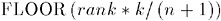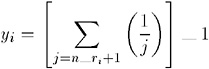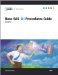# Syntax: RANK Procedure

Reminder: You can use the ATTRIB, FORMAT, LABEL, and WHERE statements. See Chapter 3, Statements with the Same Function in Multiple Procedures, on page 57 for details. You can also use any global statements. See Global Statements on page 18 for a list.

PROC RANK < option(s) >;

• BY <DESCENDING> variable-1

• < <DESCENDING> variable-n >

• <NOTSORTED>;

• VAR data-set- variables (s) ;

• RANKS new-variables(s) ;

To do this

Use this statement

Calculate a separate set of ranks for each BY group

BY

Identify a variables that contain the ranks

RANKS

Specify the variables to rank

VAR

### PROC RANK Statement

`  PROC RANK  <  option(s)  >; `

To do this

Use this option

Specify the input data set

DATA=

Create an output data set

OUT=

Specify the ranking method

Compute fractional ranks

FRACTION or NPLUS1

Partition observations into groups

GROUPS=

Compute normal scores

NORMAL=

Compute percentages

PERCENT

Compute Savage scores

SAVAGE

Reverse the order of the rankings

DESCENDING

Specify how to rank tied values

TIES=

Note: You can specify only one ranking method in a single PROC RANK step.

#### Options

DATA= SAS-data-set

• specifies the input SAS data set.

• Main discussion: Input Data Sets on page 19

• Restriction: You cannot use PROC RANK with an engine that supports concurrent access if another user is updating the data set at the same time.

DESCENDING

• reverses the direction of the ranks. With DESCENDING, the largest value receives a rank of 1, the next largest value receives a rank of 2, and so on. Otherwise, values are ranked from smallest to largest.

• Featured in: Example 1 on page 837 and Example 2 on page 839

FRACTION

• computes fractional ranks by dividing each rank by the number of observations having nonmissing values of the ranking variable.

• Alias: F

• Interaction: TIES=HIGH is the default with the FRACTION option. With TIES=HIGH, fractional ranks are considered values of a right-continuous empirical cumulative distribution function.

GROUPS= number-of-groups

• assigns group values ranging from 0 to number-of-groups minus 1. Common specifications are GROUPS=100 for percentiles, GROUPS=10 for deciles, and GROUPS=4 for quartiles. For example, GROUPS=4 partitions the original values into four groups, with the smallest values receiving, by default, a quartile value of 0 and the largest values receiving a quartile value of 3.

The formula for calculating group values is• where FLOOR is the FLOOR function, rank is the value s order rank, k is the value of GROUPS=, and n is the number of observations having nonmissing values of the ranking variable.

If the number of observations is evenly divisible by the number of groups, each group has the same number of observations, provided there are no tied values at the boundaries of the groups. Grouping observations by a variable that has many tied values can result in unbalanced groups because PROC RANK always assigns observations with the same value to the same group.

• Tip: Use DESCENDING to reverse the order of the group values.

• Featured in: Example 3 on page 841

NORMAL=BLOM TUKEY VW

• computes normal scores from the ranks. The resulting variables appear normally distributed. The formulas are

 BLOM y i = ˆ’ 1 ( r i ˆ’ 3/8)/( n +1/4) TUKEY y i = ˆ’ 1 ( r i ˆ’ 1/3)/( n +1/3) VW y i = ˆ’ 1 ( r i )/( n +1)
• where ˆ’ 1 is the inverse cumulative normal (PROBIT) function, r i is the rank of the i th observation, and n is the number of nonmissing observations for the ranking variable.

VW stands for van der Waerden. With NORMAL=VW, you can use the scores for a nonparametric location test. All three normal scores are approximations to the exact expected order statistics for the normal distribution, also called normal scores . The BLOM version appears to fit slightly better than the others (Blom 1958; Tukey 1962).

• Interaction: If you specify the TIES= option, then PROC RANK computes the normal score from the ranks based on non-tied values and applies the TIES= specification to the resulting normal score.

NPLUS1

• computes fractional ranks by dividing each rank by the denominator n +1, where n is the number of observations having nonmissing values of the ranking variable.

• Aliases: FN1, N1

• Interaction: TIES=HIGH is the default with the NPLUS1 option.

OUT= SAS-data-set

• names the output data set. If SAS-data-set does not exist, PROC RANK creates it. If you omit OUT=, the data set is named using the DATA n naming convention.

PERCENT

• divides each rank by the number of observations that have nonmissing values of the variable and multiplies the result by 100 to get a percentage.

• Alias: P

• Interaction: TIES=HIGH is the default with the PERCENT option.

• Tip: You can use PERCENT to calculate cumulative percentages, but use GROUPS=100 to compute percentiles.

SAVAGE

• computes Savage (or exponential) scores from the ranks by the following formula (Lehman 1998):TIES=HIGH LOW MEAN

• specifies how to compute normal scores or ranks for tied data values.

• HIGH

• assigns the largest of the corresponding ranks (or largest of the normal scores when NORMAL= is specified).

• LOW

• assigns the smallest of the corresponding ranks (or smallest of the normal scores when NORMAL= is specified).

• MEAN

• assigns the mean of the corresponding rank (or mean of the normal scores when NORMAL= is specified).

• Default: MEAN (unless the FRACTION option or PERCENT option is in effect).

• Interaction: If you specify the NORMAL= option, then the TIES= specification applies to the normal score, not to the rank that is used to compute the normal score.

• Featured in: Example 1 on page 837 and Example 2 on page 839

### BY Statement

Produces a separate set of ranks for each BY group.

Main discussion: BY on page 58

Featured in: Example 2 on page 839 and Example 3 on page 841

BY <DESCENDING> variable-1

• < <DESCENDING> variable-n >

• <NOTSORTED>;

#### Required Arguments

variable

• specifies the variable that the procedure uses to form BY groups. You can specify more than one variable. If you do not use the NOTSORTED option in the BY statement, the observations in the data set must either be sorted by all the variables that you specify, or they must be indexed appropriately. Variables in a BY statement are called BY variables .

#### Options

DESCENDING

• specifies that the observations are sorted in descending order by the variable that immediately follows the word DESCENDING in the BY statement.

NOTSORTED

• specifies that observations are not necessarily sorted in alphabetic or numeric order. The observations are grouped in another way, such as chronological order.

The requirement for ordering or indexing observations according to the values of BY variables is suspended for BY-group processing when you use the NOTSORTED option. In fact, the procedure does not use an index if you specify NOTSORTED. The procedure defines a BY group as a set of contiguous observations that have the same values for all BY variables. If observations with the same values for the BY variables are not contiguous, the procedure treats each contiguous set as a separate BY group.

### RANKS Statement

Creates new variables for the rank values.

Requirement: If you use the RANKS statement, you must also use the VAR statement.

Default: If you omit the RANKS statement, the rank values replace the original variable values in the output data set.

Featured in: Example 1 on page 837 and Example 2 on page 839

RANKS new-variables(s) ;

#### Required Arguments

new-variable (s)

• specifies one or more new variables that contain the ranks for the variable(s) listed in the VAR statement. The first variable listed in the RANKS statement contains the ranks for the first variable listed in the VAR statement, the second variable listed in the RANKS statement contains the ranks for the second variable listed in the VAR statement, and so forth.

### VAR Statement

Specifies the input variables.

Default: If you omit the VAR statement, PROC RANK computes ranks for all numeric variables in the input data set.

Featured in: Example 1 on page 837, Example 2 on page 839, and Example 3 on page 841

VAR data-set-variables(s) ;

#### Required Arguments

data- set-variable (s)

• specifies one or more variables for which ranks are computed.

#### Using the VAR Statement with the RANKS Statement

The VAR statement is required when you use the RANKS statement. Using these statements together creates the ranking variables named in the RANKS statement that correspond to the input variables specified in the VAR statement. If you omit the RANKS statement, the rank values replace the original values in the output data set.Base SAS 9.1 Procedures Guide, Volumes 1, 2, 3 and 4
ISBN: 1590472047
EAN: 2147483647
Year: 2004
Pages: 142